Question

# The region bounded by f(x)=−2sin(x) x=π, x=2π, and y=0 is rotated about the y-axis. Find the...

The region bounded by f(x)=−2sin(x) x=π, x=2π, and y=0 is rotated about the y-axis. Find the volume of the solid of revolution.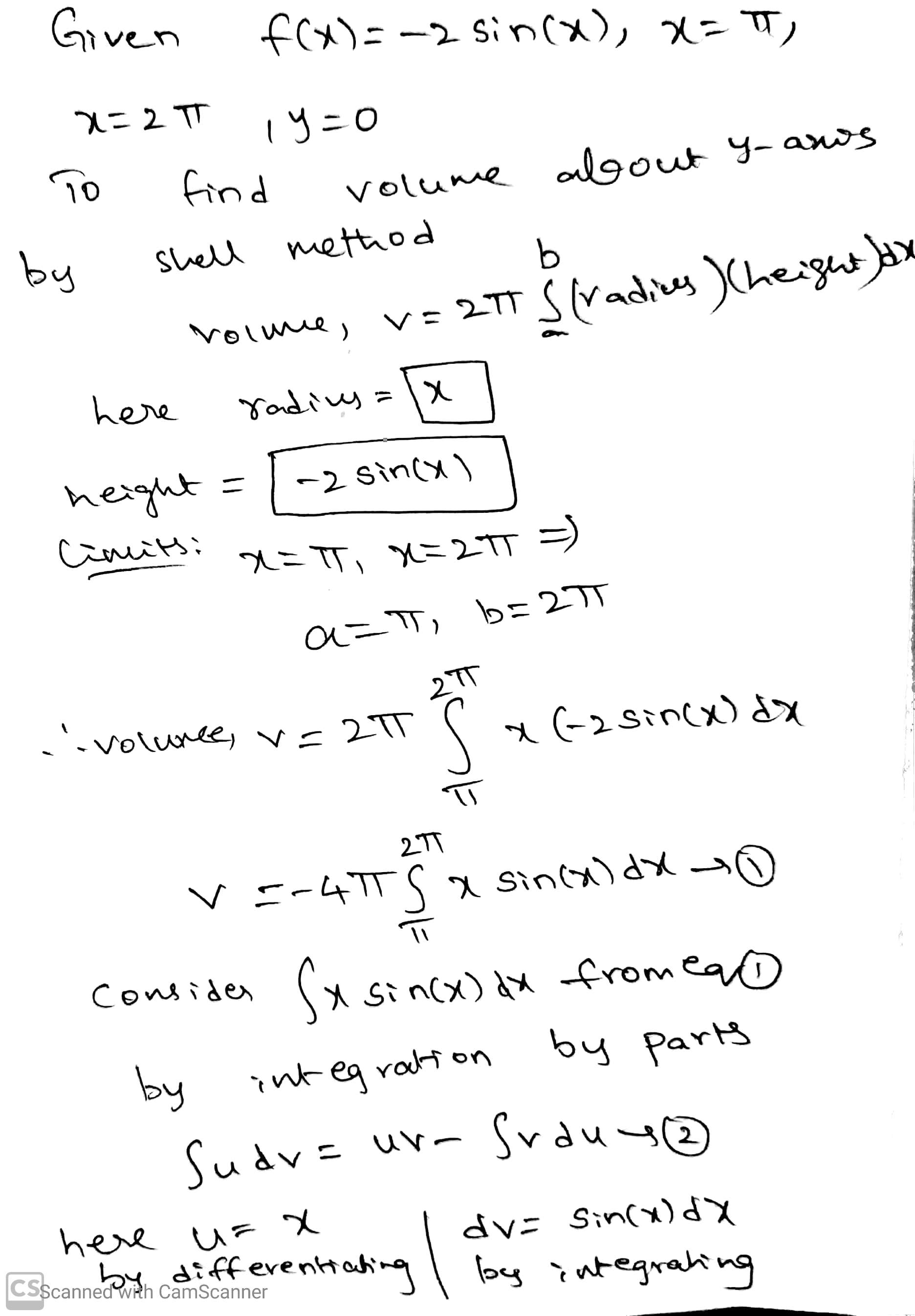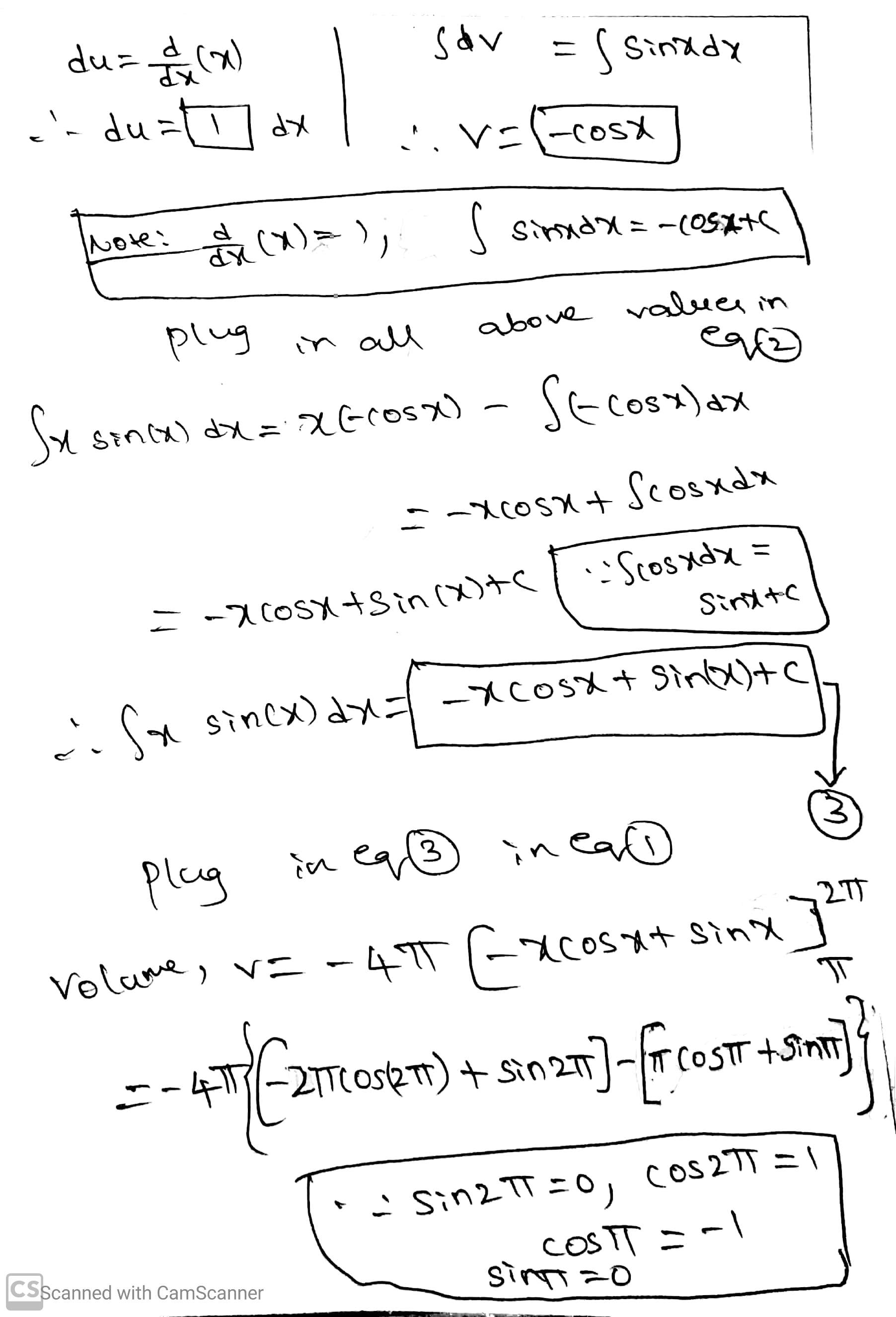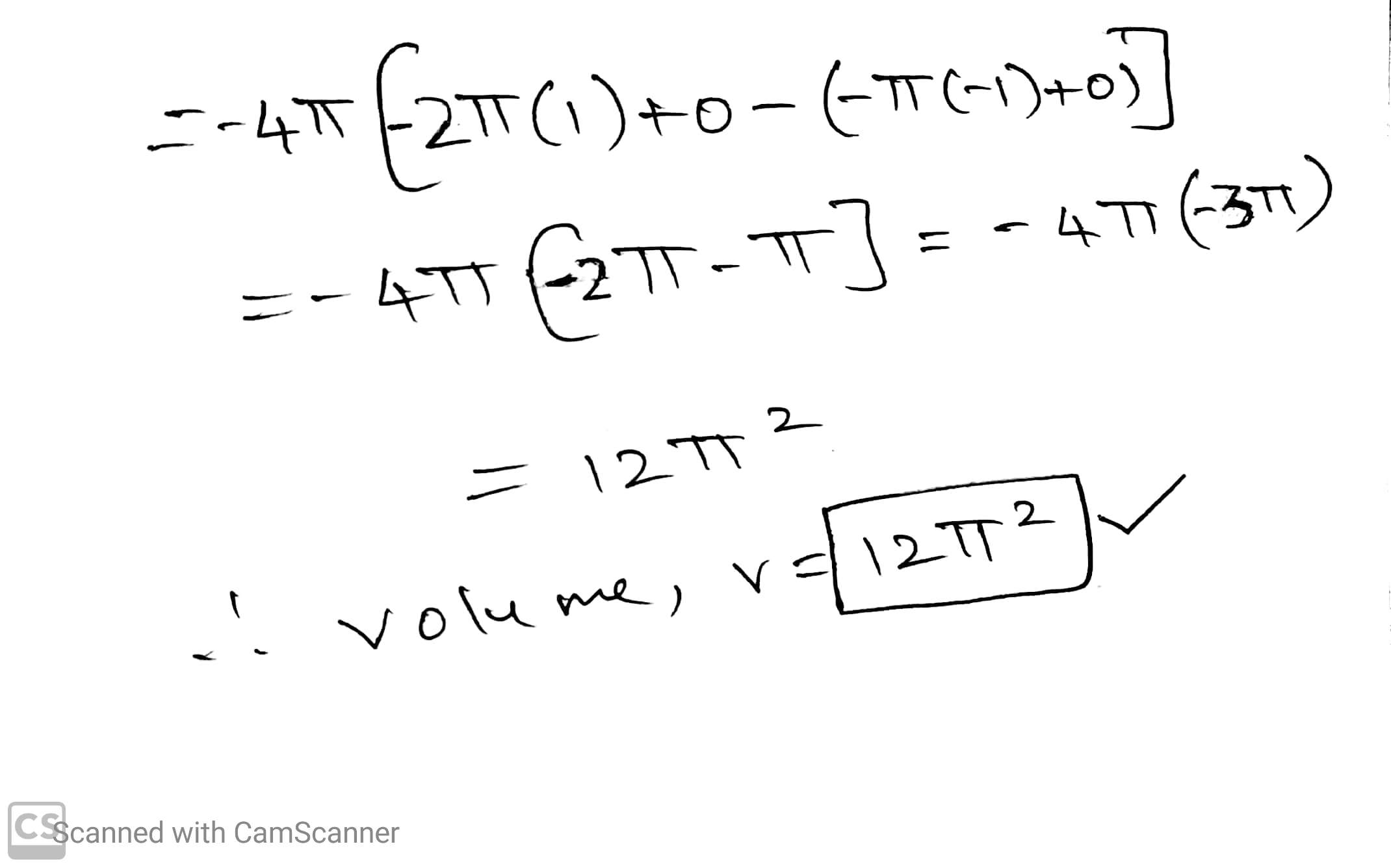#### Earn Coins

Coins can be redeemed for fabulous gifts.

Similar Homework Help Questions
• ### The region bounded by x = 25 + y x = 0, y = 5, and y = 10 is rotated about the x-axis. Find the volume of the solid of revolution Use your calculator or computer to find the answer rounded to 4 d...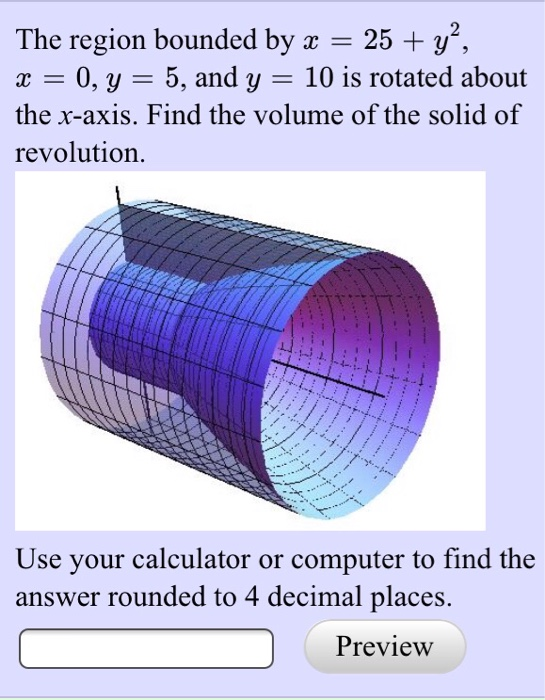The region bounded by x = 25 + y x = 0, y = 5, and y = 10 is rotated about the x-axis. Find the volume of the solid of revolution Use your calculator or computer to find the answer rounded to 4 decimal places. Preview The region bounded by x = 25 + y x = 0, y = 5, and y = 10 is rotated about the x-axis. Find the volume of the solid of revolution Use...

• ### 2) The region R in the first quadrant of the xy-plane is bounded by the curves...

2) The region R in the first quadrant of the xy-plane is bounded by the curves y=−3x^2+21x+54, x=0 and y=0. A solid S is formed by rotating R about the y-axis: the (exact) volume of S is = 3) The region R in the first quadrant of the xy-plane is bounded by the curves y=−2sin(x), x=π, x=2π and y=0. A solid S is formed by rotating R about the y-axis: the volume of S is = 4) The region bounded...

• ### all answer Sample Test 4 1575 Calculus II 1. The region bounded by the parabola y-4x-x and the x -axis is revolved about thex- axis. Find the volume of the solid. Write answer in term of π. Find...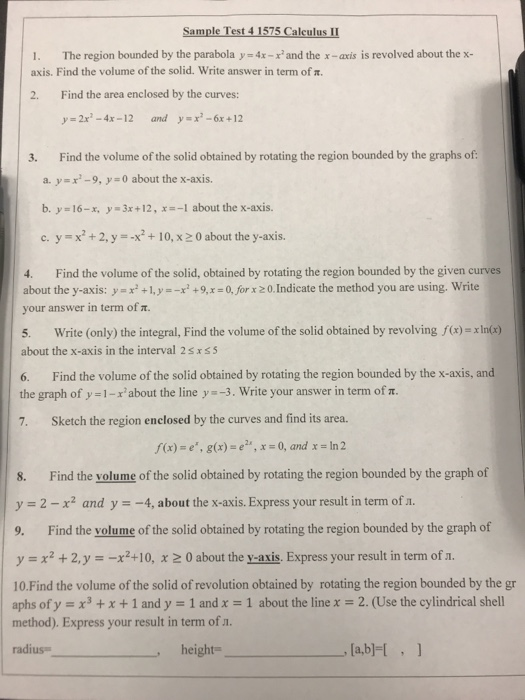all answer Sample Test 4 1575 Calculus II 1. The region bounded by the parabola y-4x-x and the x -axis is revolved about thex- axis. Find the volume of the solid. Write answer in term of π. Find the area enclosed by the curves: 2. y=2x2-4x-12 y=x2-6x+12 and 3. Find the volume of the solid obtained by rotating the region bounded by the graphs of a. y-x-9, y 0 about the x-axis. -1 about the x-axis. b. y 16-r, y-3x+...

• ### 24. The region bounded by f'(x) = -4sinx , x = 1 , x = 211...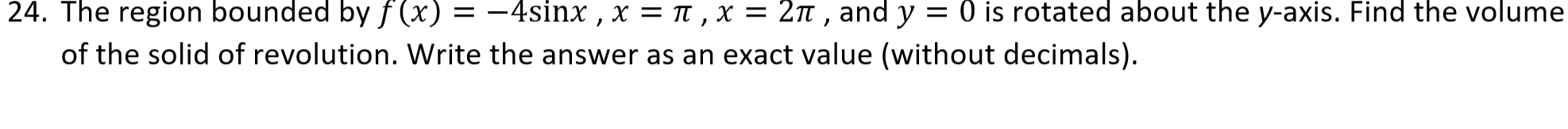24. The region bounded by f'(x) = -4sinx , x = 1 , x = 211 , and y = 0 is rotated about the y-axis. Find the volume of the solid of revolution. Write the answer as an exact value (without decimals).

• ### The region bounded by the given curves is rotated about the specified axis. Find the volume...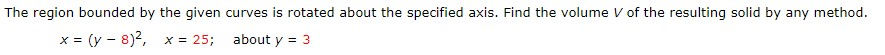The region bounded by the given curves is rotated about the specified axis. Find the volume V of the resulting solid by any method. x = (y-8)2, x = 25; about y = 3

• ### The region bounded by the given curves is rotated about the specified axis. Find the volume...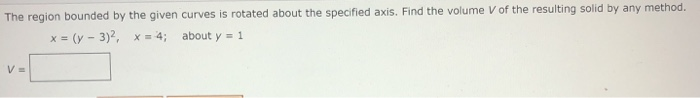The region bounded by the given curves is rotated about the specified axis. Find the volume V of the resulting solid by any method. x = (y - 3)2, x = 4; about y = 1 VE

• ### The region bounded by the given curves is rotated about the specified axis.

The region bounded by the given curves is rotated about the specified axis. Find the volume V of the resulting solid by any method y = 8, y = x +7/x, about the x = -2

• ### 4. Find the volume of the solid formed by the curves x = 1-y^4 and x= 0, and rotated about the y-axis 5. Calculate the volume of the solid obtained by rotating the region bounded by the curves y = x^2...

4. Find the volume of the solid formed by the curves x = 1-y^4 and x= 0, and rotated about the y-axis 5. Calculate the volume of the solid obtained by rotating the region bounded by the curves y = x^2, y=0, x=-2 https://gyazo.com/cedb31d3c70d20f6947f520b865a0307

• ### volumes of revolution 3) Find the volume of the solid formed by revolving the region bounded by the graphs of y- x+1, y +1, x 0, and x-1 about the x-axis. 3) Find the volume of the solid form...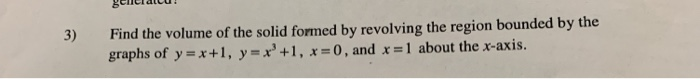volumes of revolution 3) Find the volume of the solid formed by revolving the region bounded by the graphs of y- x+1, y +1, x 0, and x-1 about the x-axis. 3) Find the volume of the solid formed by revolving the region bounded by the graphs of y- x+1, y +1, x 0, and x-1 about the x-axis.

• ### Find the volume of the solid obtained when the region bounded by the y- axisthe region bounded byy- 3a2- andy-0 is...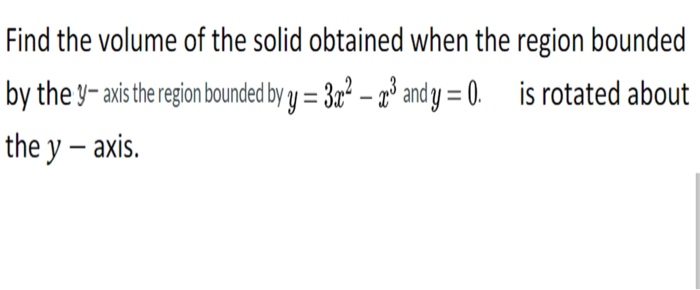Find the volume of the solid obtained when the region bounded by the y- axisthe region bounded byy- 3a2- andy-0 is rotated about the y - axis. Find the volume of the solid obtained when the region bounded by the y- axisthe region bounded byy- 3a2- andy-0 is rotated about the y - axis.